# What is Voltage, Basic Concept of Voltage For Student:

Voltage can be defined as the potential difference between the two charges points. Otherwise, electric pressure between two points, or electric potential energy per unit charge between two points.

Let us consider a charged particle +Q1 at a fixed point, E is electric field intensity of Q1 charge, consider another charge Q2 which is a little bit far away from the charge Q1 and First consider both has infinity difference between them Also the repulsion force between Q1 and Q2 is zero. Now move Q2 to a point A.

r2 is the distance between Q1 to Q2. Then some work is expanded on Q2 against the electric field E.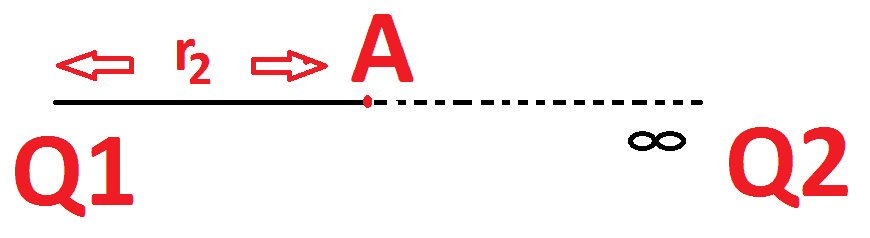The Workdone in moving charge Q2 from infinity distance to a point A can be described in terms of electric field intensity E is given by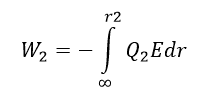Here the sign indicates the work is done against the electric field. Now the electrical potential at point A is defined as the ratio between the Work done to charge mathematically it can be expressed as.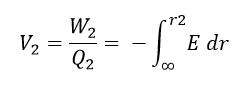Also Voltage or Electric potential can be defined as work done to move a unit of charge from infinity to a particular point. Mathematically,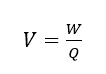For shifting one coulomb charge (6.028 x 10 ^ 18 Electrons) from infinity to a certain point in an electric field, the work done is 1 joule. And the potential at a point is 1 volt.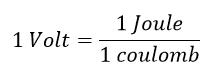Also we know the electric field due to Q1 is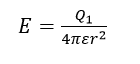Substitute on the formula of V2, Then V2 is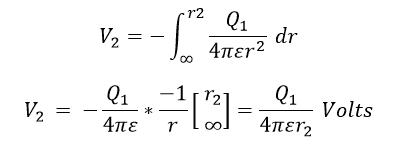Here we can consider two case:

Case 1:

Potential at a point A which is at a distance d From the Positive charge Q, The the Voltage V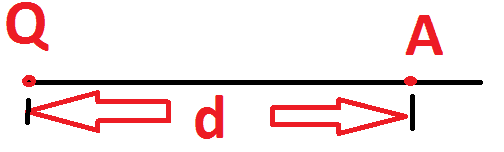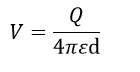Similarly let we consider another charge Q3 and the same move it from infinity to certain distance r3 and r3 is smaller than r2, the workdone in moving the charge Q3 is more. The potential at point B is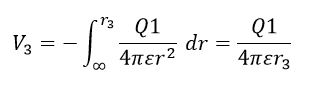Because of the potential at point B V3 is always greater than V2, if the positive charge moves from higher potential to lower potential there is some energy will be lost or spend, that is called Voltage drop.

If the unit charge moves from lower potential to higher potential, the same receives energy, that is Voltage rise.

## Basic Concept of Voltage:

Current always try to flow from high voltage and to low voltage end. This is the main concept behind in generator parallel operation (Normally it is called Synchronization). The greater the voltage, the greater the flow of electrical current (Charge carrier pass a fixed quantity at per unit of time) through a conducting or semiconducting medium for a given resistance to the flow.

Voltage is the electrical force that causes free electrons to move from one atom to another whenever the passive loads are connected, general passive loads are resistor, inductor, or other electronic loads. Example: Just as water needs some pressure to force it through a pipe, electrical current needs some force to make it flow.

“Volts” is the measure of “electrical pressure” that causes current flow. Voltage is sometimes referred to as the measure of a potential difference between two points along a conductor

Learn More:   LED Light Power Consumption Calculation & LED Energy Bill Calculator

Voltage is normally denoted by a letter V or E. V stands for Voltage and E stands for Emf. The SI unit of Voltage is Volt (V)

### One Volt:

As per Ohms law, one Volt can be derived as one unit of charge (one Coulomb) carriers pass through a one-ohm resistance in one second. Here one coulomb is equal to 6.028 x 10 ^ 18 electrons.

### Types of Voltage:

According to its direction Voltage can be separated by two types;

1) Alternating Voltage

2) Direct Voltage

Here alternating voltage changes its polarity periodically, the periodical action is measured in terms of frequency, SI unit of frequency is Hertz (Hz) (one Hz is equal to one cycle per second), Alternating Voltage is mainly used for utility appliance.

Here Direct Voltage do not change its direction, it constantly maintains its polarity. Simply, Direct voltage is the potential difference between the two terminals of electro chemical cell (typically positive and negative).